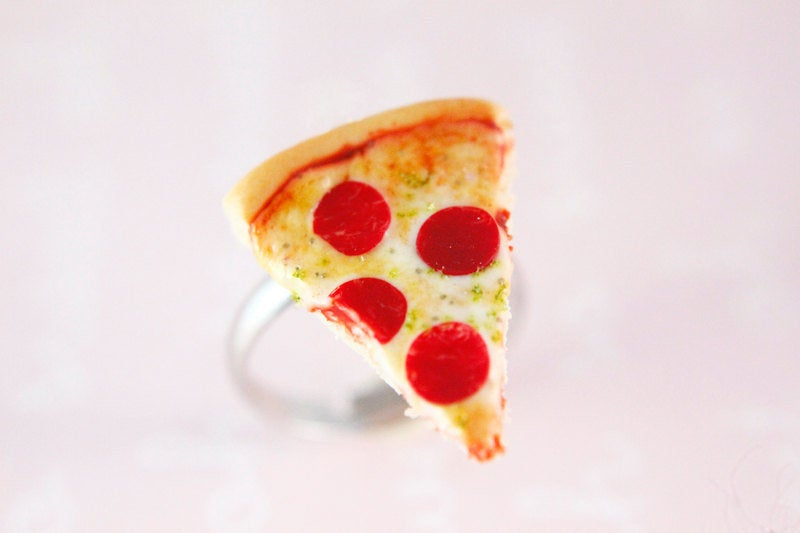# The Mathematical Reason You Should Always Order The Bigger Pizza

Math has never been so fun.

Ever downsized your pizza order to save a little cash? A new video from AsapSCIENCE proves you’ve been doing it wrong.

It all comes down to a basic geometric equation from your nightmares your high school math class. The area of a circle is equal to pi times the radius squared.

According to that equation, a basic eight-inch pizza has an area of 50 square inches. While you might think a sixteen-inch pizza is twice as big as an eight-inch pizza, given that the diameter is two times bigger, the math tells us otherwise.

A sixteen-inch pizza has an area of 200 square inches ― four times as large as the eight-inch pizza. But as AsapSCIENCE’s video points out, the difference in cost between a small pizza and an extra large pizza is rarely two times more expensive, never mind four times as expensive.

The math is clear. You get the most bang for your buck when you order the larger pizza. Pro-tip: save your leftovers and reheat them in a skillet for next-day pizza glory.

Remember that time you asked your math teacher when you would ever use geometric equations in the real world? Consider this their “booyah” moment.

## Before You Go